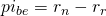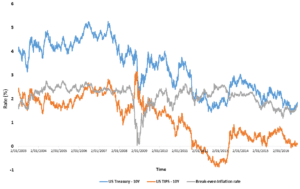# Inflation expectations

Inflation expectations refer to people’s expectations about the future rate of inflation. There are several ways in which the expected inflation rate can be determined. On this page we discuss and illustrate how inflations expectations can be inferred from surveys and break-even inflation rates.

## Determining Inflation expectations

There are two ways in which economists try to determine inflation expectations. The first approach consists of surveys. In this case, people are asked about their inflation expectations. There are a number of disadvantages to using this approach. First, inflation expecations are only available at a very low frequency, i.e. after every survey. Second, the approach is very costly and time-consuming. It is also prone to errors. A second, more commonly used approach, relies on bond prices. In the next section, we show how you can use bond yields to infer expected inflation.

## Calculation break-even inflation rate

To calculate inflation inspectations, market participants often make use of break-even inflation rates. The most well-known break-even inflation rate is the FRED’s 10 year break-even inflation rate, which is widely used by market participants. To infer the break-even inflation rate, we need to collect the nominal yield on a fixed-rate bond and the real yield on an inflation-linked bond that has the same maturity. Then, the break-even inflation rate formula equalswhere rn is the nominal yield and rr is the real yield. The above inflation formula shows that the break-even inflation rate is simple the difference between the yield on a nominal fixed-rate bond and the real yield on an identical inflation-linked bond.

## Example break-even inflation

The figure below plots the 10 year break-even inflation rate using US TIPS and US treasury data. The corresponding break-even inflation rate, which is the difference between the nominal and real yield reported in the figure, reflects market participants’ inflation expectations over the next 10 years.## Summary

We explain the concept of inflation expecations and how we can easily infer these expectations from nominal and real bond yields. Subtracting the real yield on a TIPS from the nominal bond yield (which an easily be done using Excel) provides an easy proxy of inflation expectations.